# RD Sharma Solutions Chapter 5 Trigonometric Ratios Exercise 5.1 Class 10 Maths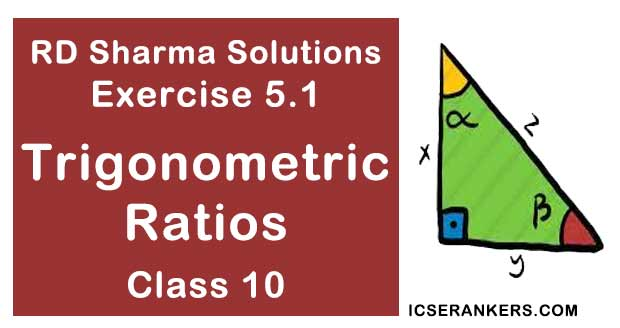Chapter Name RD Sharma Chapter 5 Trigonometric Ratios Book Name RD Sharma Mathematics for Class 10 Other Exercises Exercise 5.2Exercise 5.3 Related Study NCERT Solutions for Class 10 Maths

### Exercise 5.1 Solutions

1. In each of the following one of the six trigonometric ratios is given. Find the values of the other trigonometric ratios.

Solution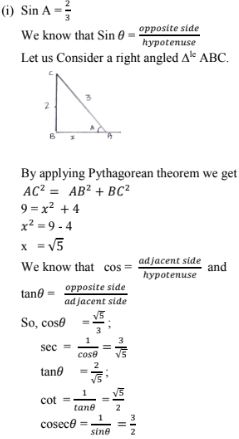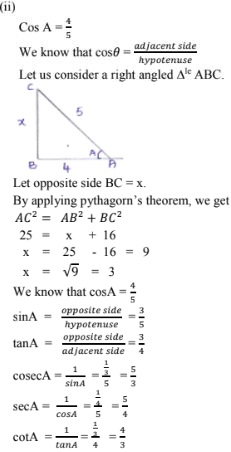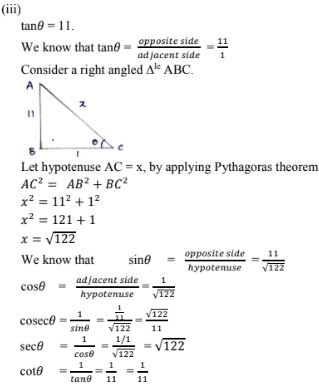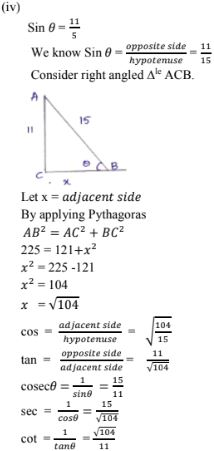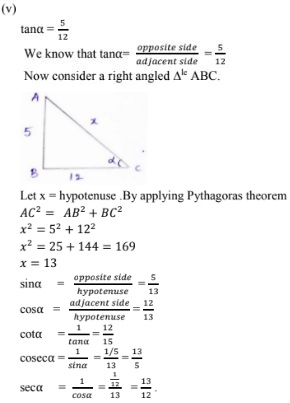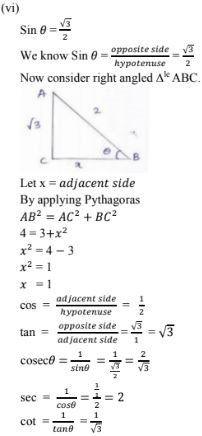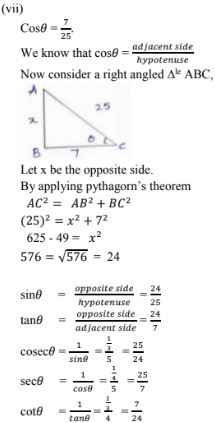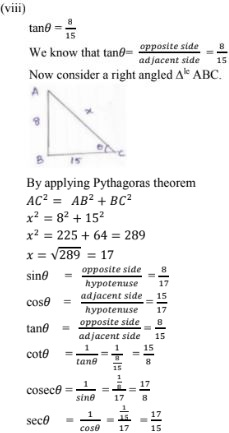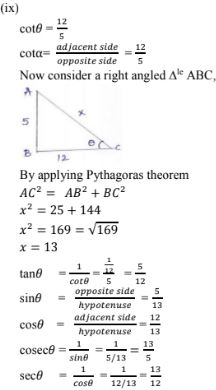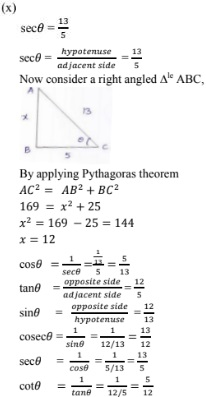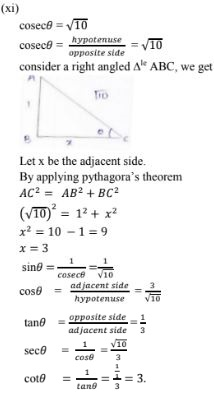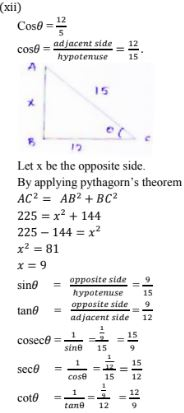2. In a ΔABC, right angled at B, AB = 24 cm, BC = 7cm. Determine
(i) SinA, CosA
(ii) SinC, CosC
Solution
ΔABCis right angled at B
AB = 24cm, BC = 7cm.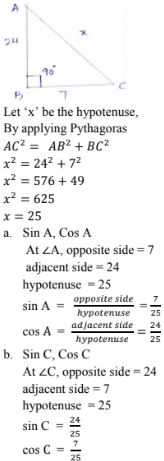3. In Fig below, Find tan P and cot R. Is tan P = cot R?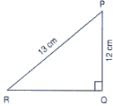Solution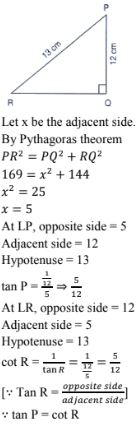4. If sin A = 9/41,  compute cosA and tanA
Solution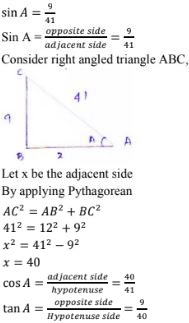5.Given 15 cotA = 8, find Sin A and secA.
Solution

15 cot A = 8, find Sin A and sec A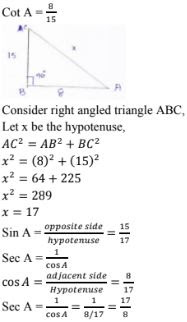6. In ΔPQR, right angled at Q, PQ = 4 cm and RQ = 3 cm. Find the values of sin P, sin R, sec P and sec R.
Solution

ΔPQR, right angled at Q.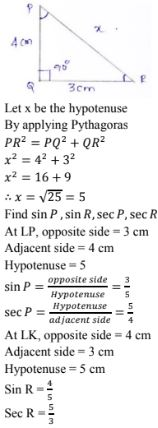7. If cot θ =7/8, evaluate :
(i) [(1 + sinθ)(1 - sinθ)]/[(1 + cosθ)(1 - cosθ)
(ii) Cot2θ
Solution8. If 3cotA = 4, check whether (1 - tan2A)/(1 + tan2A) = cos2A - sin2A or not.
Solution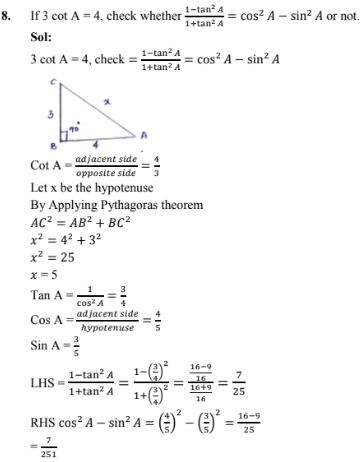9. If tan θ = a/b, find the value of (cosθ + sinθ)/(cosθ - sinθ).
Solution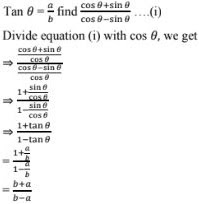10. If 3 tanθ = 4, find the value of (4cosθ - sinθ)/(2cosθ + sinθ).
Solution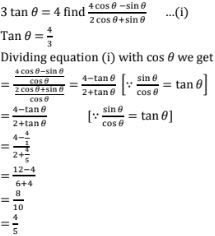11. If 3cotθ = 2, find the value of = (4sinθ - 3cosθ)/(2sinθ + 6sinθ)
Solution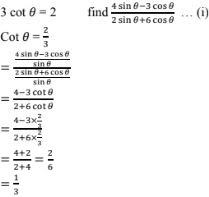12. If tanθ = a/b, prove that (a sinθ - b cosθ)/(a sinθ + b cosθ) = (a2 - b2)/(a2 + b2).
Solution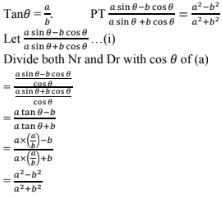13. If secθ = 13/5, show that (2cosθ - 3cosθ)/(4sinθ - 9cosθ) = 3
Solution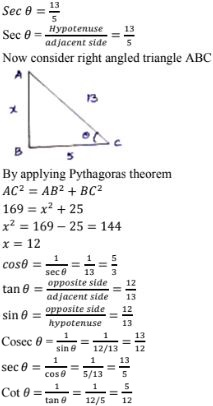14. If cosθ = 12/13, show that sinθ (1 - tanθ) = 35/156 .
Solution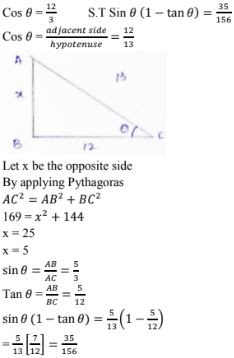15. If cotθ = 1/√3, show that  (1 - cos2θ)/(2 - sin2θ) = 3/5 .
Solution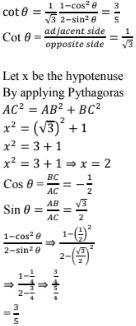16. If tanθ = 1/√7
(cosec2θ  - sec2θ)/(cosec2θ + sec2θ) = 3/4
Solution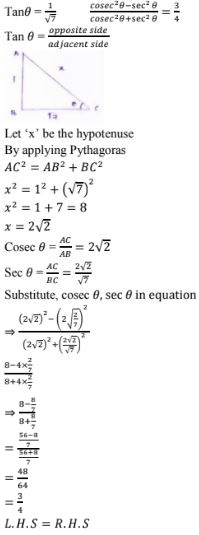17. If Sinθ = 12/13 find (sin2θ - cos2θ)/(2 sinθ cosθ) × 1/tan2θ
Solution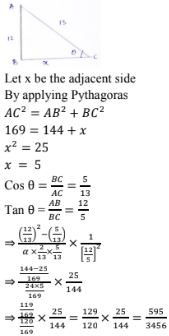18.  If secθ = 5/4 , find the value of (sinθ - 2cosθ)/(tanθ - cotθ)
Solution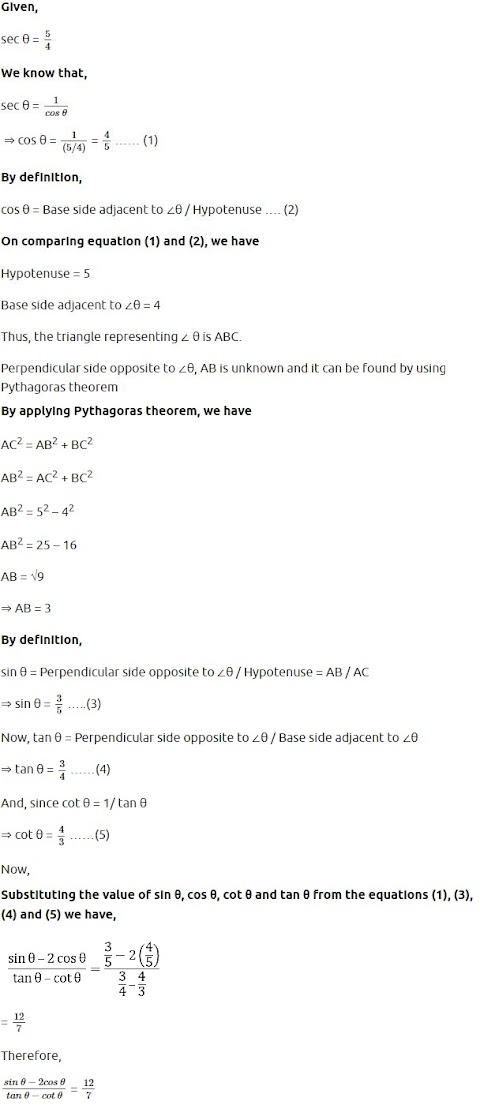19. Tanθ = 12/13
Find (2sinθ cosθ)/(cos2θ - sin2θ)
Solution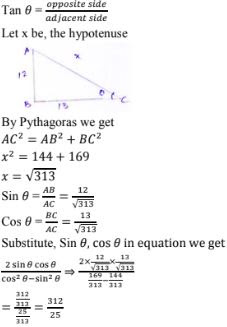21. If cosθ = 3/5, find the value of  (sinθ - 1/tanθ)/2tanθ .
Solution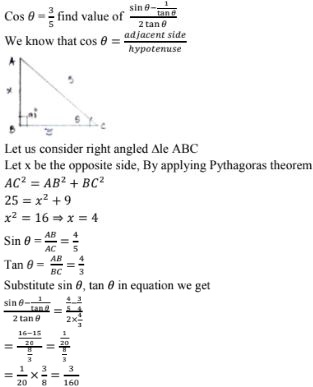22. If sinθ = 3/5, evaluate  (cosθ - 1/tanθ)/2cotθ
Solution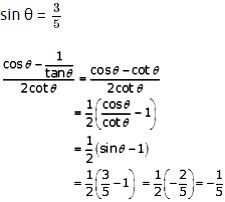23. If sec A = 5/4, verify that (3sinA - 4sin3A)/(4cos3A - 3cos A) = (3tanA - tan3A)/(1 - 3tan2A).
Solution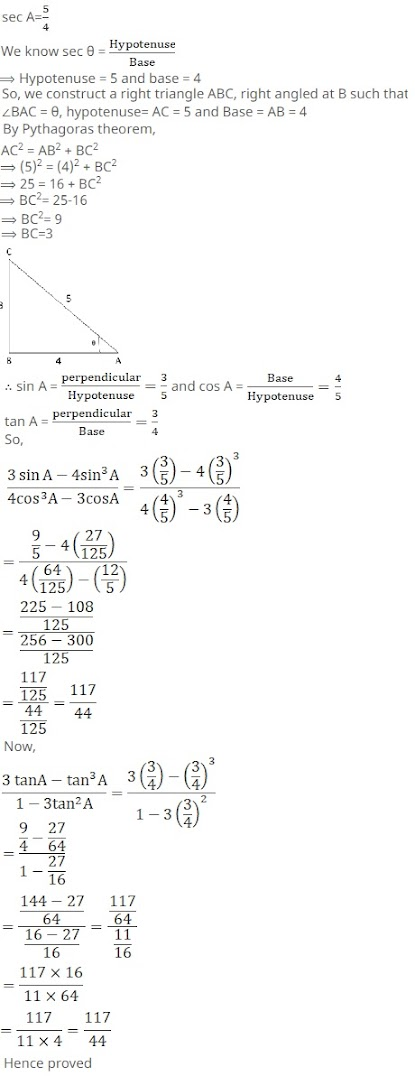24. If sec A = 17/8, verify that (3 - 4sin2A)/(4cos2A - 3) = (3 - tan2A)/(1 - 3tan2A)
Solution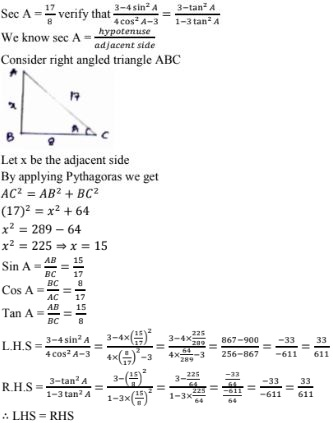25. If cot θ = 3/4, prove that √(secθ - cosecθ)/(secθ + cosecθ) = 1/√7
Solution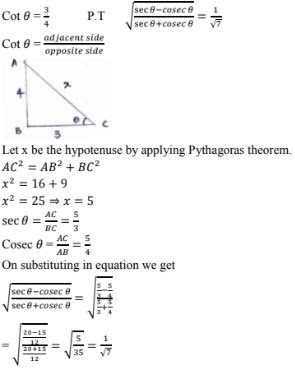27. If tanθθ = 24/7, find that sin θ + cos θ
Solution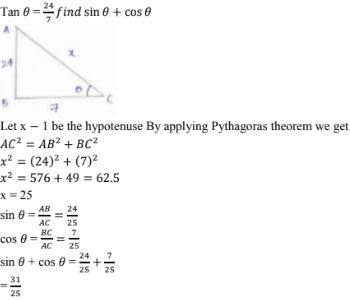28. If sin θ = a/b, find sec θ + tanθ in terms of a and b.
Solution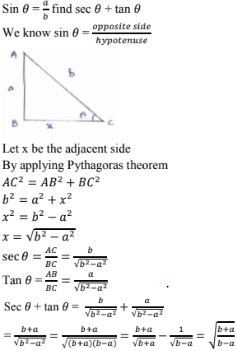29.  If 8 tan A = 15, find  sinA - cosA.
Solution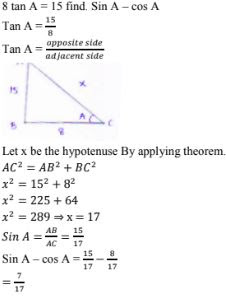30. If 3cosθ - 4sinθ = 2cosθ + sinθ Find tanθ .
Solution

3cosθ - 2cosθ = 4sinθ + sinθ find tanθ
3cosθ - 2cosθ = sinθ + 4sinθ
cosθ = 5sinθ
Dividing both side by use we get
cosθ/cosθ = 5sinθ/cosθ
1 = 5tanθ
⇒ tanθ = 1

31.  If tanθ = 20/21, show that (1- sinθ + cosθ)/(1 + sinθ + cosθ) = 3/7
Solution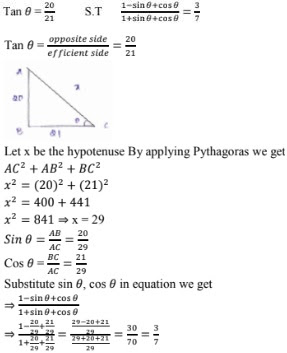32. If Cosec A = 2 find 1/tanA + sinA/(1 + cosA)
Solution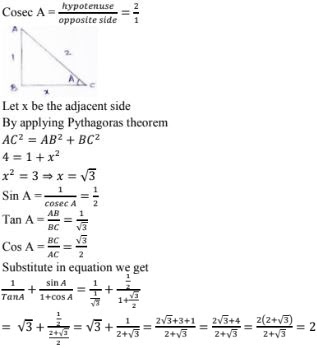33. If ∠A and ∠B are acute angles such that cos A = cos B, then show that ∠A = ∠B.
Solution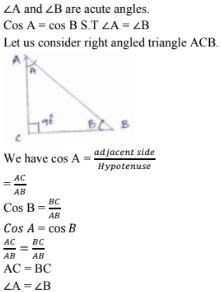34. If ∠A and ∠P are acute angles such that tan A = tan P, then show that ∠A = ∠P.
Solution

A and P are acute angle tan A = tan P
S.T.     ∠A = ∠P
Let us consider right angled triangle ACP,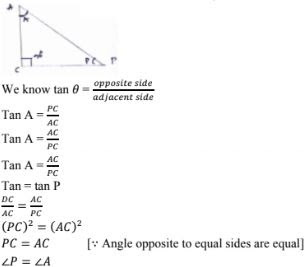35. In a ΔABC, right angled at A, if tan C = √3, find the value of sin B cos C + cos B sin C .
Solution
In a Δle  ABC right angled at A tan C = √3
Find sin B cos C + cos B sin C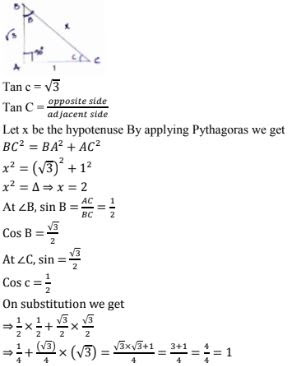36. State whether the following are true or false. Justify your answer.
(i) The value of tan A is always less than I.
(ii)  Sec A = 12/5 for some value of angle A.
(iii) Cos A is the abbreviation used for the cosecant of angle A.
(iv) Sin θ = 4/3 for some angle θ.
Solution

(a) Tan A ∠1
Value of tan A at 45° i.e., tan 45 = 1
As value of A increases to 90°
Tan A becomes infinite
So given statement is false.

(b) Sec A = 12/5 for some value of angle of
M - I
Sec A = 2.4
Sec A > 1
So given statement is True
M - II
For sec A = 12/5
For sec A = 12/5 we get adjacent side = 13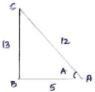We get a right angle Δle
Subtending 9i at B.
So, given statement is true.
(c)  Cos A is the abbreviation used for cosecant of angle A.
The given statement is false
∴ Cos A is abbreviation used for cos of angle A but not for cosecant of angle A.
(d) Cot A is the product of cot A and A
Given statement is false
∵ cot A is co - tangent of angle A and co - tangent of angle A = (adjacent side)/(opposite side)
(e)  Sin θ = 4/3  for some angle θ
Given statement is false
Since value of sin θ is less than (or) equal to one. Here value of sin θ exceeds one, so given statement is false.